This video was brought to you by GeneralPAC.com, making power systems Intuitive, Open and Free for Everyone, Everywhere. Consider subscribing and supporting through patreon.com/GeneralPAC. This is a mechanism for you to support us financially so we can continue making high quality power system video tutorials. Our corporate sponsor for this topic is AllumiaX.com from Seattle, Washington. Contact them for industrial and commercial power system studies.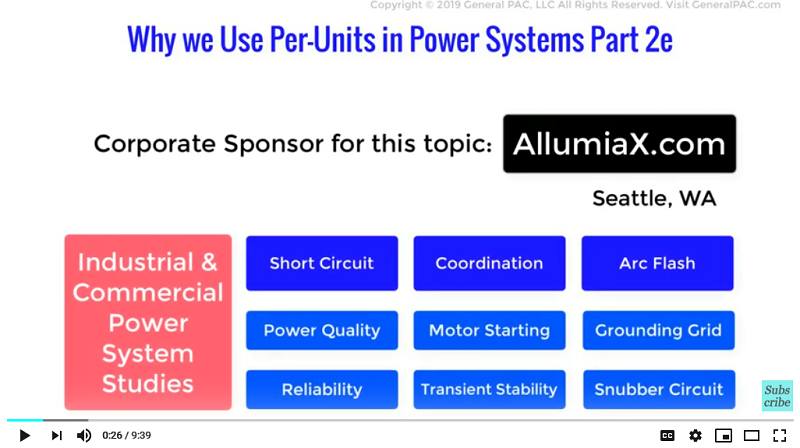Why we use Per-Unit Systems, Part 2e.

In this video, we will demonstrate the fourth and final advantage of using Per-unit systems in calculations.

Let’s start with the basics, in order to perform power system analysis of a three-phase system, we have to perform per phase and line to line conversions that involves a factor of √3. There are formulas to achieve this, but it is quite prone to errors and miscalculations due to an irrational number being present i.e. √3. Also this under-root three factor can be an annoying eyesore in hand calculations. However, when the impedances, voltages and currents in a three-phase system are transformed into their per unit equivalents, the need of complex calculations is eliminated. The use of √3 is reduced to one or two steps. To polish the idea, let’s solve an example from the book Electric Power Systems by B.M. Weedy (Fifth Edition).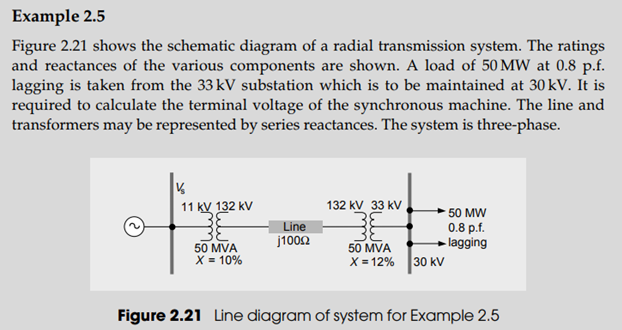So, now we have to calculate Vs that is the voltage being supplied by the machine to the entire power system. Let’s now analyze the system. We are given with two transformers which means we have to calculate their impedances. There is a transmission line, so we have to calculate the impedance offered by the transmission line. And then, there is load that has to be maintained on a specific voltage.

First step is to identify the base power. Since both the transformers have apparent power = 50MVA, we’ll choose this as the base power for the whole system.

Now, here is one new thing that is to be seen. There is something marked as X under the two transformers. This X is the reactance of the transformer that is shown in percentage. We take this percentage as the per unit value of the reactance offered by the component in a power system. So, we conclude that the reactance offered in per unit by transformer 1 and transformer 2 are j0.1 p.u and j0.12 p.u respectively.

XT1 = j0.1 p.u

XT2 = j0.12 p.u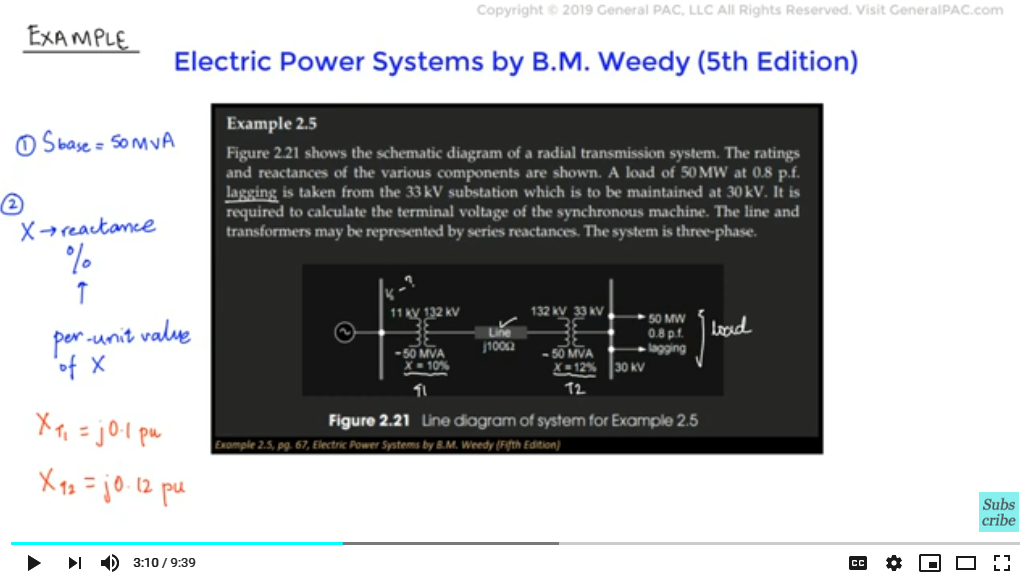Since we know the per unit impedances of the transformers, we will now calculate the per-unit impedance of the transmission line.

For the base impedance of the transmission line

Z Tx,base =
VTx,base 2 / STx,base
=
(132 KV)2 / 50 * 106 VA
= 348.5 Ω

After that, we simply calculate the per unit impedance of the transmission line by:

Z Tx,p.u =
ZTx / ZTx,base
=
j100 Ω / 348.5 Ω
= j0.287 p.u

So, the series impedance in this power system will be the sum of all the impedances calculated meaning:

Ztotal = XT1,pu + XT2,pu + ZTx,p.u = j0.1 + j0.12 + j0.287 = j0.507 p.u

Next, we determine the current that should flow in the power system and into the load if the load has to be maintained at 30kV. We have derived the formula that relates the base power and base voltage in Part 1d of this series. The formula is:

S = √3 × V × I – this is for unity Power Factor.

We will rearrange this formula and calculate the current flowing through the load and add the Power Factor correction.

We will rearrange this formula and calculate the current flowing through the load and add the Power Factor correction.

I =
S / √3 × V × cosθ
=
50 × 106 VA / √3 × 30kV × 0.8
= 1203 A

Cos θ is added because the load is operating at a power factor and this leads us to a value of 1203 Amps angle negative 36.86 degrees. We have a VVsnegative angle because the current lages the voltage by 36.86 degrees.

We will now calculate the base current in the power system and the load:

Ibase =
Sbase / √3 × Vbase
=
50 × 106 VA / √3 × 33× 103 V
874.8 A

Calculating the per unit value of current:

Ip.u =
I / Ibase
=
1203A ∠ - 36.86° / 874.8 A
= 1.375 ∠ - 36.86° p.u

Calculating the per unit voltage at the load:

V / Vbase
=
30 kV / 33 kV
= 0.91 p.u

Now, we apply Kirchoff’s law and calculate the total voltage at the source in per units:

Vs,p.u = Ip.u × Ztotal + Vload,p.u

However, we also need to take into account of the lagging PF 0.80. According to the problem statement, the current lags voltage by PF of 0.80. Which means the current lags voltage by 36.86 degrees.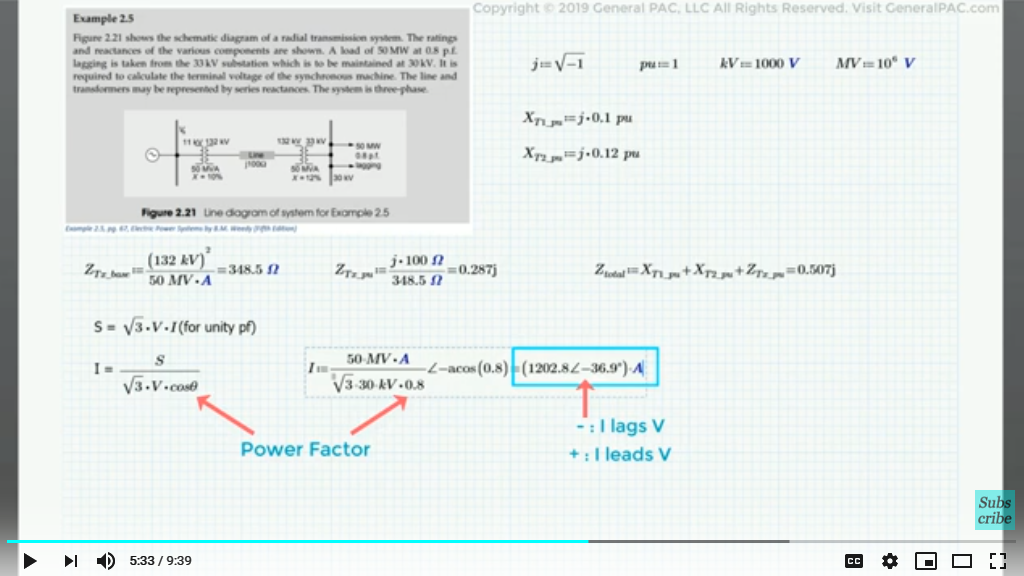Vs,p.u = (1.375 ∠ -36.86° × j0.507) + 0.91pu

Vs,p.u = 1.328 + j0.558 p.u = 1.44 ∠ 22.79° p.u

Or:

So, we have the per unit value of the terminal voltage and we know the formula to calculate the actual voltage at the generating source.

Vs,p.u =
Vs / Vs,base

Rearranging the equation and solving for Vs:

Vs = Vs,p.u × Vs,base

The base voltage at the generating source is 11kV, that is the primary voltage of transformer 1. We can ignore the angle resulting in:

Vs = 1.44pu × 11kV

Vs = 15.84 kV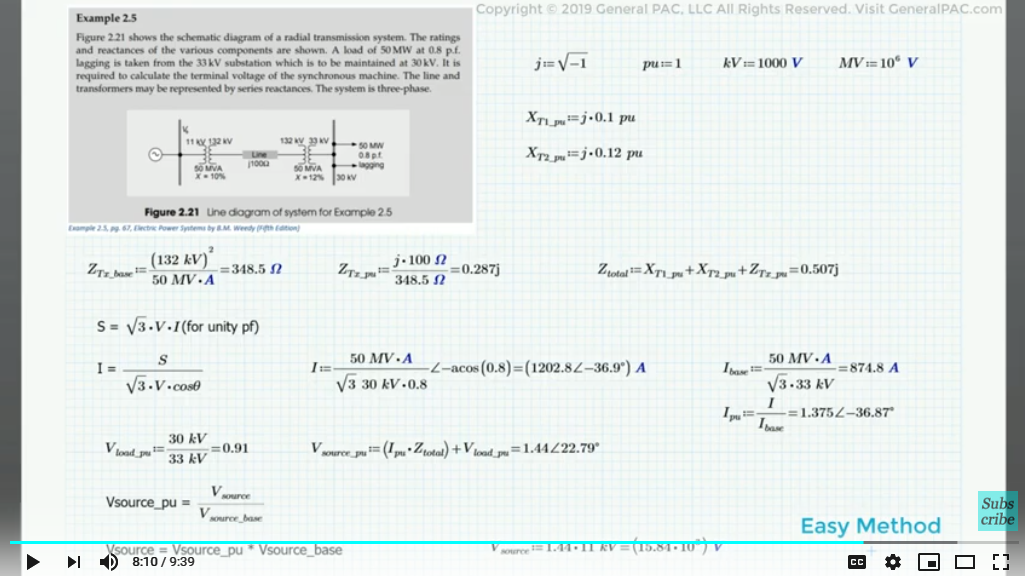Finally, we have solved this example using a very easy method of per-unit calculations. Notice that we have not used √3 once we converted the system into per-unit equivalent. It was just used in the two steps to calculate the base values. Therefore, if we want to analyze a three-phase system, we could simply calculate the per unit values and eliminate the need of √3.

This video concludes the second part of the series Power System Analysis. In this part we saw the four major advantages of using per-unit systems in the analysis of power systems. If we notice one thing here, these all processes were manual ones. We would solve these examples using pen and paper and we are sure that per-unit calculations ease our work. Just imagine if per-unit calculations can reduce our time and effort while doing them by hand, what effect would they have on a machine! We can conveniently claim that if we use computational methods, the significance of per-unit systems will increase.

In the next part we will perform some further derivations and solve more complex problems.

We hope, you have a continued interest in this topic and series as a student or professional. We also hope you find this content useful and enlightening. Please consider subscribing to GeneralPac.com or becoming a patron on patreon.com.

GeneralPAC.com. Making Power Systems Intuitive and free to everyone, anywhere, everywhere.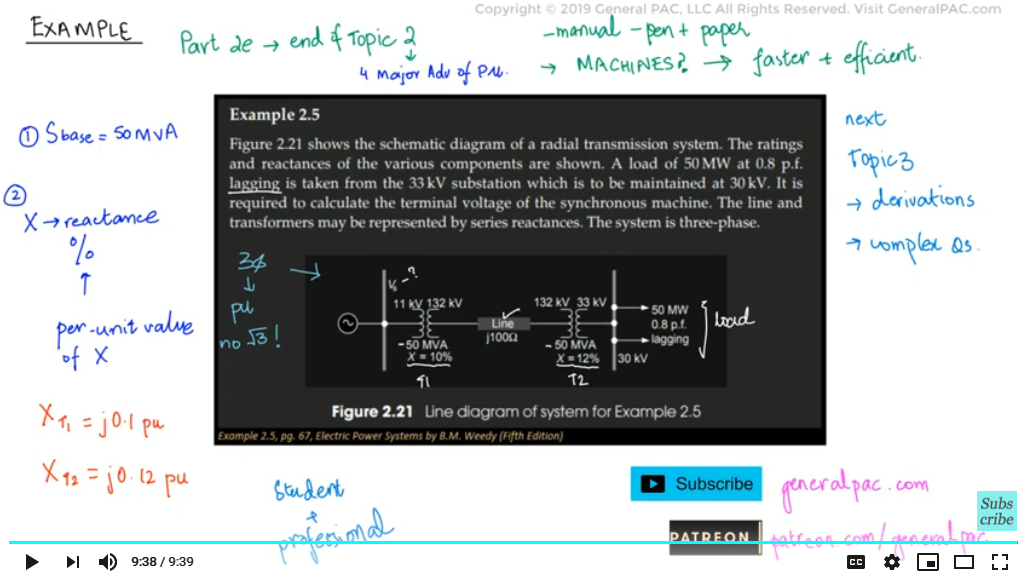#### Greetings from the GeneralPAC Team!

We make high-quality Power Systems Video Tutorials on complex topics that are free and open to everyone!  Thank you so much for supporting us through Patreon so we can continue doing good and valuable work.

What is Patreon and why do we use it?

Patreon is a fantastic portal that allows our fans and community to make monthly contribution (like Netflix subscription) so we can continue creating high-quality power systems video tutorials. In return, you get access to incredible perks like voting on future topics, getting your questions answered, access to VIP Q/A webinars with the creators of GeneralPAC, and much more! We THANK YOU for supporting us

Why do we need your support?

An incredible amount of time and effort is needed to develop high-quality video tutorials. Each video (Part 1 for example) takes approximately 10 hours to complete which includes learning the concept ourselves, brainstorming creative ways to teach and explain the concepts, writing the script, audio recording, video recording, and editing. It's no wonder why Hundreds-of-Thousands of people have watched, liked, subscribed, and left positive comments on Youtube channel. Your support truly makes all the difference.